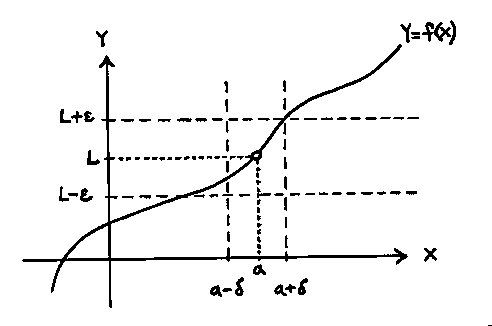# Definition of a limit

• Chas3down
In summary, the limit definition states that there exists a positive delta such that for all values of x within the range of the delta, ##|f(x) - L| < \epsilon##.f

lim (x - 4) = 2
as x -> 6

eps > 0
delta > 0

No eps given

## The Attempt at a Solution

Not sure how to apply equation..
Usually I would do

I assumed you would do..

6 - eps < x - 4 - 2 < 6 + eps
12 - eps < x < 12 + eps

So, it would be min, (12-eps,12+eps)

But what do I do from here?

Last edited:

lim (x - 4) = 2
as x -> 6

eps > 0
delta > 0

No eps given

## The Attempt at a Solution

Not sure how to apply equation..
Usually I would do

I assumed you would do..

6 - eps < x - 4 - 2 < 6 + eps
12 - eps < x < 12 + eps

So, it would be min, (12-eps,12+eps)

But what do I do from here?

You seem to be confused with the core concept of this question ( The limit definition ). Here's an easy way to remember the definition in symbols:

$$\forall \epsilon > 0, \exists \delta(\epsilon) > 0 \space | \space 0 < |x-a| < \delta \Rightarrow |f(x) - L| < \epsilon$$

It basically reads : Forall positive epsilon, there exists a positive delta in terms of epsilon such that we can make ##f(x)## as close to ##L## as we like. A more in depth explanation of what's going on is below.

Considering ##0 < |x-a| < \delta##, we know that ##|x-a|## must always be positive, so we never actually consider what happens at ##x=a##, only what happens as we approach it. That's where ##|x-a| < \delta## comes into play.

Expanding we get ##-\delta < x-a < \delta## and then ##x + \delta > a > x -\delta##.

So there exists a ##\delta > 0## such that ##a## is bounded between ##x + \delta## and ##x -\delta## and we can only arbitrarily get close to ##a##.

Using this, what does it say about ##|f(x) - L| < \epsilon##?

Well first, let's consider ##-\epsilon < f(x) - L < \epsilon## which yields ##f(x) + \epsilon > L > f(x) - \epsilon##. So ##\forall \epsilon > 0## we can make ##f(x)## as close to ##L## as we like. How close do we need to be you might ask? Sufficiently close.

What defines sufficiently close ( You probably hear this a lot )? Well, ##f(x)## varies according to the values of ##x##. How far away ##x## is from ##a## depends on ##\delta##. So the values of how far ##f(x)## is away from ##L## ( which change according to ##\epsilon## ) will also vary according to ##\delta##. Hence ##\epsilon## will change according to the ##\delta## we choose.

So in conclusion we know we can choose a ##\delta(\epsilon)## as to make ##f(x)## as close to ##L## as we like.

So as for your question, start with the ##|f(x) - L|## portion and massage it a bit to get it into a suitable form.

Last edited:
I just want to add this picture to supplement Zondrina explanation..

Thanks a lot to both of you! When I get back from class I will thoroughly read through all of it and hopefully able to conceptually understand it.

EDIT: I'm an idiot..

i forgot delta = eps in linear functions with a slope of one ;D

Last edited: